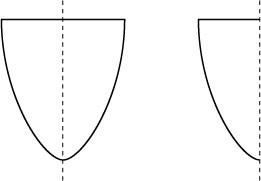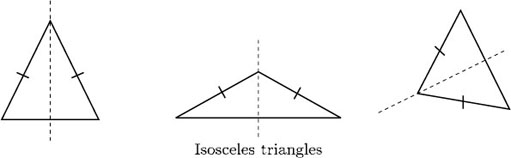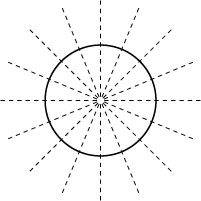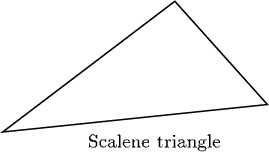Science, Maths & Technology

### Become an OU studentGeometry

Start this free course now. Just create an account and sign in. Enrol and complete the course for a free statement of participation or digital badge if available.

# 2.6 Line symmetry

Look at the shapes below. The symmetry of the shape on the left and its relationship to the shape on the right can be thought of in two ways:

• Fold the left-hand shape along the central line. Then one side lies exactly on top of the other, and gives the shape on the right.

• Imagine a mirror placed along the central dotted line. The reflection in the mirror gives the other half of the shape.This type of symmetry is called line symmetry.

Any isosceles triangle has line symmetry.The dashed lines represent lines of symmetry, and each shape is said to be symmetrical about this line.

The following all have line symmetry:

A shape can have more than one line of symmetry. Thus a rectangle has two lines of symmetry, an equilateral triangle has three lines of symmetry, and a square has four.

A circle has an infinite number of lines of symmetry since it can be folded about any diameter. Only eight of the possible lines of symmetry are indicated below.Some shapes, such as a scalene triangle, have no lines of symmetry – it is not possible to fold the shape about a line so that the two halves fit exactly on top of one another.